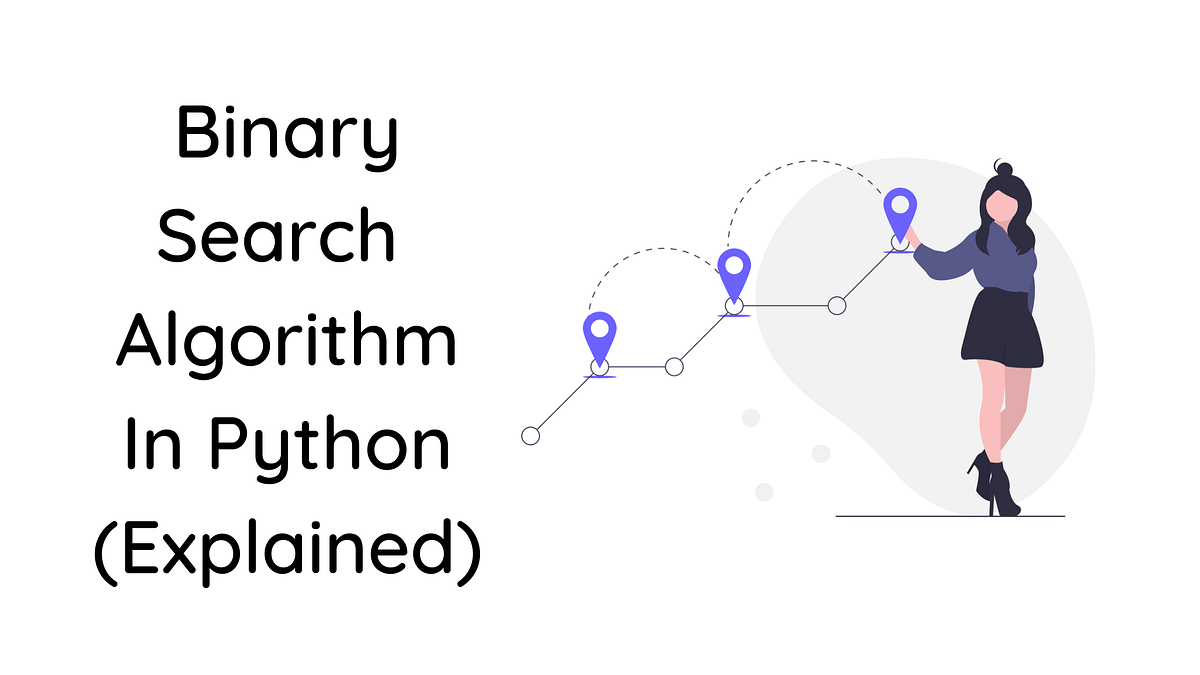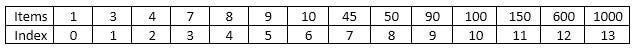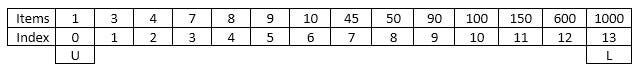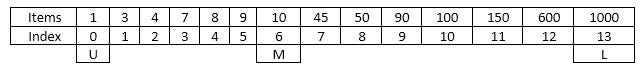# Binary Search Algorithm in Python (Explained)Binary search also known as half-interval search, logarithmic search is an algorithm used in computers to find the position of a value in a sorted array.

Binary search also known as half-interval search, *logarithmic search *is an algorithm used in computers to find the position of a value in a sorted array.

Bubble sortLinear search, Quick sort are some of the other type of sorting algorithm available. In this article we are going to learn about the searching algorithm known as Binary search.

Binary search is little bit more advanced and have more accurate results when compared with linear search and bubble sort algorithms.

So let’s assume that you have a list with 10000 items in it. The item you are looking for is in the 9000th place. If you implement the linear search algorithm in this scenario it will take much time to give you the result. Because the algorithm needs check each and every item in the list.

So how can we write our code to show the result quickly? Here’s how….

We have a list with the following items.The List

So first we need to specify the Lower bound (L) and Upper bound (U). Lower bound is the first index of the list and upper bound is the last index of the list.specifying the lower bound and upper bound

Once you finish assigning these bounds you have to find a mix index (M).

So what is mid index? As name implies it is the middle position between lower and upper bounds. So we can say that,

Mid Index = (Lower bound + Upper bound)/2

In my case, Mid Index = (0 + 13)/2 = 6Mid Index

In here we are doing integer division not float division. Therefore 13/2 = 6 not 6.5.

Now let’s specify the item that we need to find from our list. Let’s say we need to find number “90” from our list.

OK now the mid value we got is 6. The value of index 6 in our list is 10.

Now we have to check whether if mid value is matching the item that we are searching. (10 ≠90) Since they are not matching we need to change the lower bound or the upper bound. How do we know which one to change? Here’s how…

You have to check the value you are searching for is smaller or bigger than the mid value. If the value is smaller change upper bound and mid value becomes the new upper bound. If value is greater change lower bound and mid value becomes new lower bound.

## Binary Search Algorithm in Python | Data Structure | Searching Algorithm

Binary Search a sorted array or list by repeatedly dividing the search interval in half. Begin with an interval covering the whole array. If the value of the...

## Evaluating Performance on Search Algorithms in Python and Rust

Speed in search algo in Python and Rust? Ever wondered if the search algorithms that you have learnt in high school or college/university, would perform differently in different programming languages?

## Guide to Python Programming Language

Guide to Python Programming Language

## Binary Search Recursive Method in Python | Recursive Function | Searching Algorithm

Binary Search a sorted array or list by repeatedly dividing the search interval in half. Begin with an interval covering the whole array. If the value of the...

## Jump Search Algorithm in Python | Data Structure | Searching Algorithm

Jump Search is a searching algorithm for sorted array or list. The basic idea is to check fewer elements by jumping ahead by fixed steps or skipping some ele...